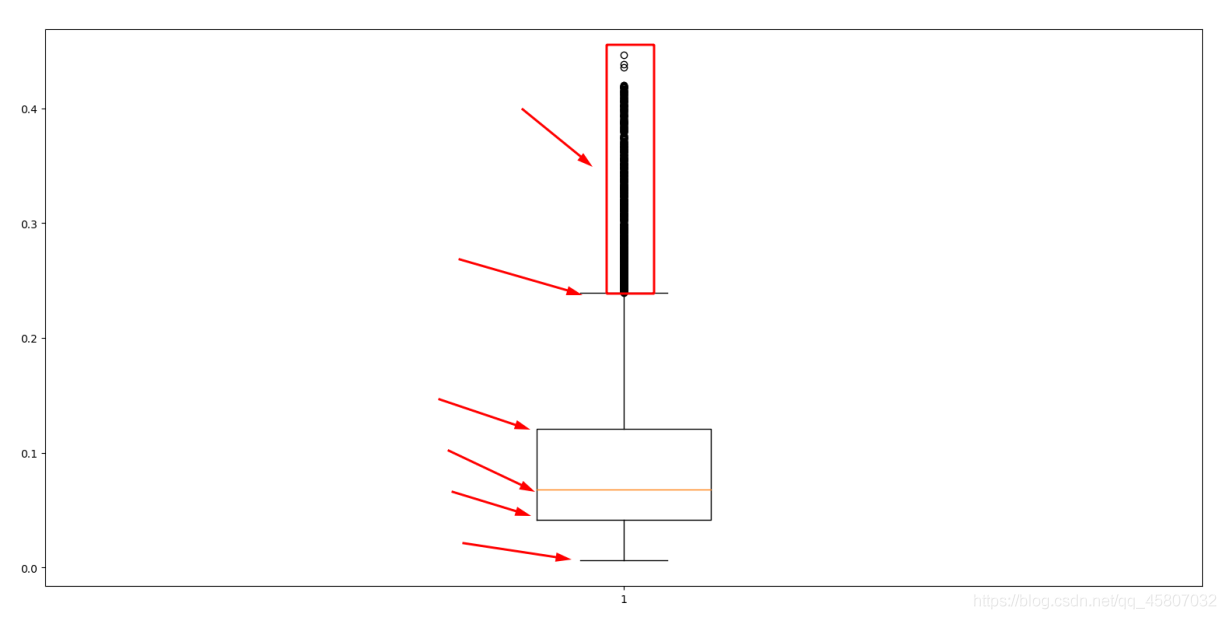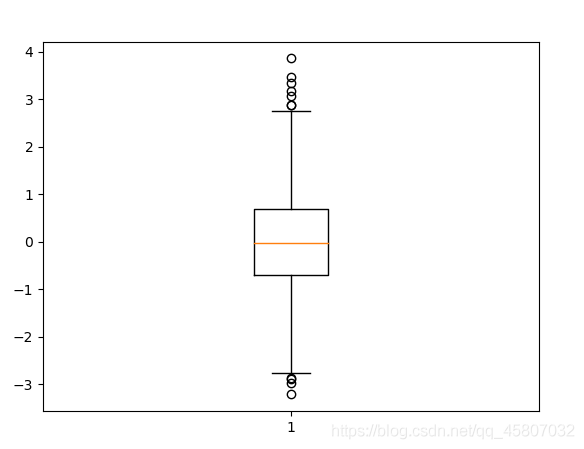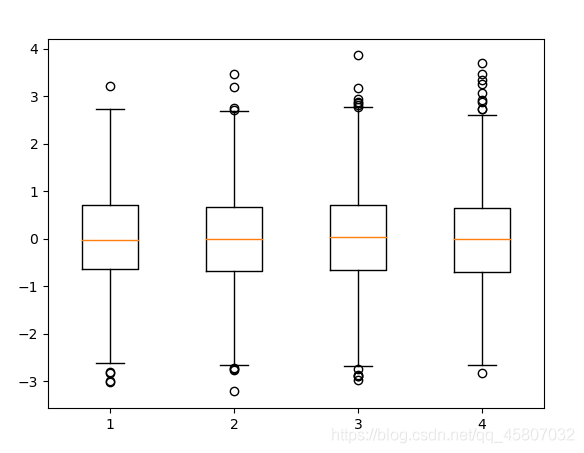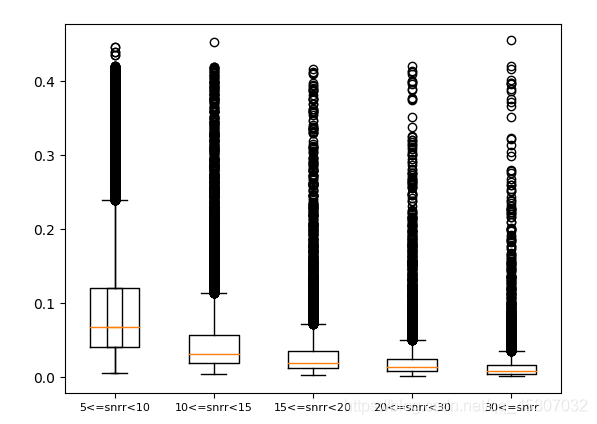# Python使用plt.boxplot()函数绘制箱图、常用方法以及含义详解

## 1. 箱图含义## 2.计算方法

Q1：中位数将所有数据分成两部分，最小值到中位数的部分按取中位数的方法取中位数作为Q1。

Q3：同Q1取法，取中位数到最大值的中位数。

IQR(四分位数间距)=Q3-Q1

## 3.绘图

### 3.1 绘制单个箱图

```import matplotlib.pyplot as plt
import numpy as np

#生成data数据
np.random.seed(100)
data = np.random.normal(size=(1000,),loc=0,scale=1)

# 绘图
plt.boxplot(data)
plt.show()
```### 3.2 绘制多个箱图

```import matplotlib.pyplot as plt
import numpy as np

np.random.seed(100)
data = np.random.normal(size=(1000,4),loc=0,scale=1)

plt.boxplot(data)

plt.show()
```### 3.3实战

```def plt_box_iamge(df):
"""
snrr的五个范围为[5,10)、[10,15)、[15,20)、[20,30)、[30-)，按照五个snrr范围计算对应redchi的箱图
:param df:包含snrr以及redchi的csv数据（dataFrame）。
:return:
"""
# 根据snrr范围对redchi进行筛选。
df1 = df.loc[df['lam_snrr'] >= 5]
redchi_1 = df1.loc[df1['lam_snrr'] < 10].redchi

df2 = df.loc[df['lam_snrr'] >= 10]
redchi_2 = df2.loc[df2['lam_snrr'] < 15].redchi

df3 = df.loc[df['lam_snrr'] >= 15]
redchi_3 = df3.loc[df3['lam_snrr'] < 20].redchi

df4 = df.loc[df['lam_snrr'] >= 20]
redchi_4 = df4.loc[df4['lam_snrr'] < 30].redchi

redchi_5 = df.loc[df['lam_snrr'] >= 30].redchi
# 绘图
ax = plt.subplot()
ax.boxplot([redchi_1, redchi_2, redchi_3, redchi_4, redchi_5])
# 设置轴坐标值刻度的标签
ax.set_xticklabels(['5<=snrr<10', '10<=snrr<15', '15<=snrr<20', '20<=snrr<30', '30<=snrr'], fontsize=8)
#	保存图片
plt.savefig('./images/box.jpg')
plt.show()

if __name__ == '__main__':
df_sc = screening(df)  # 筛选数据 (lamost数据应该在正常值范围内，不然因为数值差过大会导致绘制不出图像!)
plt_box_iamge(df_sc)
```### 3.3 参数详解

```plt.boxplot(x,                      # x：指定要绘制箱图的数据
notch=None,           # notch：是否是凹口的形式展现箱线图，默认非凹口
sym=None,              # sym：指定异常点的形状，默认为+号显示
vert=None,              # vert：是否需要将箱线图垂直摆放，默认垂直摆放
whis=None,             # whis：指定上下须与上下四分位的距离，默认为1.5倍的四分位差
positions=None,   # positions：指定箱线图的位置，默认为[0,1,2…]
widths=None,         # widths：指定箱线图的宽度，默认为0.5
patch_artist=None,        # patch_artist：是否填充箱体的颜色
meanline=None,             # meanline：是否用线的形式表示均值，默认用点来表示
showmeans=None,       # showmeans：是否显示均值，默认不显示
showcaps=None,           # showcaps：是否显示箱线图顶端和末端的两条线，默认显示
showbox=None,             # showbox：是否显示箱线图的箱体，默认显示
showfliers=None,          # showfliers：是否显示异常值，默认显示
boxprops=None,           # boxprops：设置箱体的属性，如边框色，填充色等
labels=None,                  # labels：为箱线图添加标签，类似于图例的作用
flierprops=None,          # filerprops：设置异常值的属性，如异常点的形状、大小、填充色等
medianprops=None,   # medianprops：设置中位数的属性，如线的类型、粗细等
meanprops=None,       # meanprops：设置均值的属性，如点的大小、颜色等
capprops=None,           # capprops：设置箱线图顶端和末端线条的属性，如颜色、粗细等
whiskerprops=None)   # whiskerprops：设置须的属性，如颜色、粗细、线的类型等
```

### 3.4 常用方法

```import matplotlib.pyplot as plt
import numpy as np

np.random.seed(100)
data = np.random.normal(size=(1000,4),loc=0,scale=1)

ax = plt.subplot()
ax.boxplot(data)                                 # 绘图
ax.set_xlim([0,5])                               # 设置x轴值的范围  rotation=30
# ax.set_xticks()  							      # 自定义x轴的值
ax.set_xlabel("xlabel")                  # 设置x轴的标签
ax.set_xticklabels(['A','B','C','D'],  rotation=30,fontsize=10)   # 设置x轴坐标值的标签 旋转角度 字体大小
ax.set_title("xcy")       					  # 设置图像标题
ax.legend(labels= ['A','B','C','D'],loc='best',)  # 增加图例
ax.text(x=0.2 , y=3.5 , s="test" ,fontsize=12)   # 增加注

plt.show()```

matplotlib官方文档

## 总结

0
0

0 条回复（回复会通过微信通知作者）

（微信扫码即可登录，无需注册）